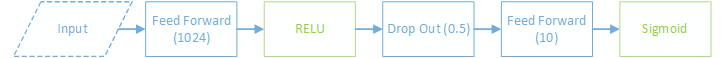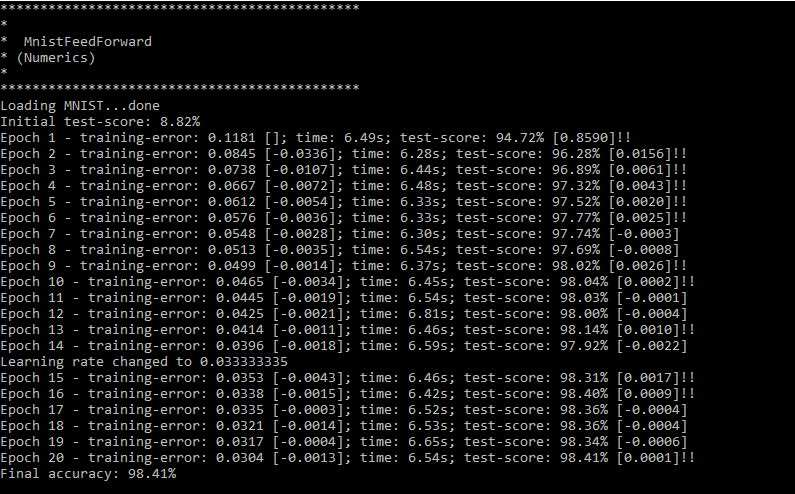# Training a vanilla feed forward Neural Network on images of handwritten digits.

## Motivation

The MNIST data set is a classic handwritten digit recognition data set. This tutorial shows how you can use Bright Wire to train a vanilla feed forward neural network to get a classification accuracy of 98%.

Bright Wire includes a helper class for loading the MNIST data, which consists of two sets of labelled image data (one for test and the other for training).

Each image is stored as 784 pixels with an intensity ranging from 0 to 255 for each value. The Mnist helper class creates vectors of length 784 and scales each value between 0 and 1.

## Network DesignSince our input layer is a feature vector of length 784 (one feature for each pixel) and the output is a one hot encoded vector of length 10 (if the training sample is an image of a three then the output vector will be 0010000000) we just need to choose the network dimensions in the middle.

A single hidden layer of size 1024 produces reasonable results, so the network ends up as 784x1024x10 with a dropout layer in between the first and second layers. The training rate is reduced after 15 epochs.

```public ExecutionGraphModel? TrainingFeedForwardNeuralNetwork(
uint hiddenLayerSize = 1024,
uint numIterations = 20,
float trainingRate = 0.1f,
uint batchSize = 128
) {
var graph = Training.Context.CreateGraphFactory();
var trainingData = graph.CreateDataSource(Training);

// one hot encoding uses the index of the output vector's maximum value as the classification label
var errorMetric = graph.ErrorMetric.OneHotEncoding;

// configure the network properties
graph.CurrentPropertySet
.Use(graph.GaussianWeightInitialisation(false, 0.1f, GaussianVarianceCalibration.SquareRoot2N))
;

// create the training engine and schedule a training rate change
var engine = graph.CreateTrainingEngine(trainingData, trainingRate, batchSize);
engine.LearningContext.ScheduleLearningRate(Convert.ToUInt32(numIterations * 0.75), trainingRate / 3);

// create the network
graph.Connect(engine)
;

// train the network, saving the model on each improvement
ExecutionGraphModel? bestGraph = null;
var testData = trainingData.CloneWith(Test);
engine.Train(numIterations, testData, errorMetric, model => bestGraph = model.Graph);

// export the final model and execute it on the training set
var executionEngine = graph.CreateEngine(bestGraph ?? engine.Graph);
var output = executionEngine.Execute(testData);
Console.WriteLine(\$"Final accuracy: {output.Average(o => o.CalculateError(errorMetric)):P2}");

return bestGraph;
}```

## Results

The classifier reaches a final accuracy of 98.41%.## Complete Source Code

View the complete source on GitHub